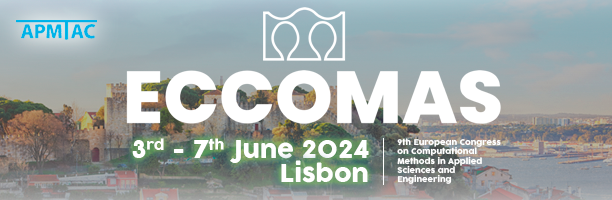## ECCOMAS 2024## MS171 - Electromagnetic Problems Arising in Industry: Modelling and Numerical Techniques

Organized by: I. Cortés (Eindhoven University of Technology, Netherlands), D. Gómez (USC and CITMAga, Spain) and S. Schoeps (Technische Universität Darmstadt, Germany)
Keywords: computational electromagnetism, discretization techniques, multi-physics, optimization
Numerical simulation in electromagnetism has undergone a relevant development in recent years since it represents a key tool in the design of devices and processes, as well as in obtaining improvements in the operation of existing prototypes. Many industrial applications involve electromagnetic problems which are often coupled with other physical models that require the solution of a coupled multiphysics problem. Therefore, it is essential to have suitable and efficient numerical schemes to approximate the electromagnetic submodel derived from Maxwell's equations. Furthermore, nowadays the numerical solution of electromagnetic goes frequently accompanied with numerical control and optimization; thus, the development of efficient algorithms is crucial to get an affordable optimization model from a computational point of view. In the literature we can distinguish two great approaches in the research field of electromagnetism: development of new models and algorithms and mathematical and numerical analysis of the models to give a theoretical support to the numerical techniques. Both approaches have provided relevant results both in the applied mathematics and in the electric engineering fields. In this context, the aim of the proposed minisymposium is to offer a forum for the discussion of recent advances in computational electromagnetism with a special focus in industrial applications. The minisymposium will try to cover several topics: techniques in modelling and discretization, multiphysics approaches, coupling between distributed and lumped models, numerical optimization, material modelling, mathematical and numerical analysis, etc. This minisymposium yields in the framework of ECMI's Special Interest Group MSOEE (Modeling, Simulation and Optimization in Electrical Engineering).